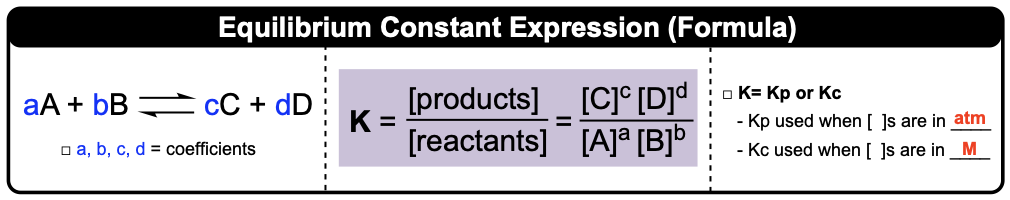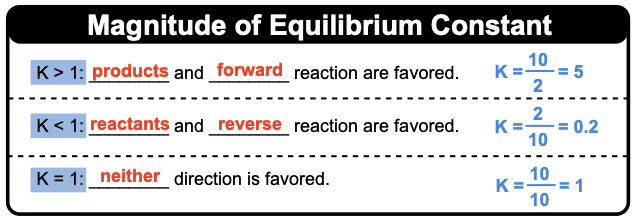Clutch Prep is now a part of Pearson
Ch.14 - Chemical EquilibriumWorksheetSee all chapters

# Equilibrium Constant (K)

See all sections
Sections
Intro to Chemical Equilibrium
Equilibrium Constant (K)
Equilibrium Constant Calculations
Kp and Kc
Using Hess's Law To Determine K
Calculating K For Overall Reaction
Le Chatelier's Principle
ICE Charts
Reaction Quotient

Equilibrium Constant (K) specifies the favored direction of a reaction.

###### Equilibrium Constant

Concept #1: Equilibrium Constant (K) Concept 1

Concept #2: Equilibrium Constant ExpressionsSolids and pure liquids are excluded from Equilibrium Expressions.

Example #1: What is the equilibrium constant expression for the following reaction:

2 HgO (s) + H2O (l) + 2 Cl(g) ⇌  2 HOCl (aq) + HgO (s) + HgCl(s)

Practice: Provide K expression for the reverse of the following reaction:

Fe2O3(s) + 3 H2(g) ⇌ 2 Fe(s) + 3 H2O(g)

Concept #3: Magnitude of Equilibrium ConstantMagnitude of Equilibrium Constant (K) indicates how far right or left a reaction lies at equilibrium. This allows us to determine if more products or reactants exist at equilibrium.

Example #2: When this reaction comes to an equilibrium, which will be higher in pressure, reactants or products?

2 CH4 (g) + 2 H2S (g) ⇌ CS2 (g) + 4 H2 (g) Kp = 1.3 x 103

a) reactants b) products  c) neither d) impossible to estimate

Practice: Which of the reactions is likely to produce more O2 at equilibrium at 25°C?

Practice: Consider the reaction A(g) ⇌ B(g) + C(g), with kforward of 5.7 x 10–2 and kreverse of 3.8 x 10–4. Which would be greater at equilibrium, partial pressure of A or partial pressures of B and C?